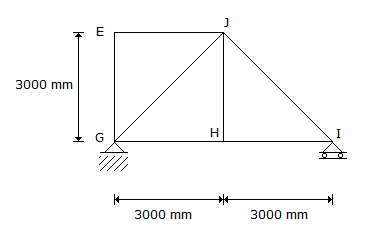# Civil Engineering - GATE Exam Questions - Discussion

### Discussion :: GATE Exam Questions - Section 2 (Q.No.49)

49.

The members EJ and IJ of steel truss shown in the figure below are subjected to a temperature rise of 30° C. The coefficient of thermal expansion of steel is 0.000012 per °C per unit length. The displacement (mm) of joint E relative to joint H along the direction HE of the truss, is[A]. 0.255 [B]. 0.589 [C]. 0.764 [D]. 1.026

Explanation:

No answer description available for this question.

 Sathya said: (Sep 23, 2015) How it will come?

 Revathi said: (Sep 23, 2016) What is the formula?

 A- Hidar said: (Aug 14, 2017) Stresses due to thermal expansion = Esteel *α * T = 2.10*10^5 * 0.000012 * 30 = 75.6 Mpa. Displacement between two point E, H is diagonal JG which has length = 4.24 m. Displacement = F*L/AE = stresses * (L/E) = 75.60 MPA * 4240 / 2.1 * 10^5 = 1.5264 mm in Diagonal which expansion tow joint E,H 1.52/2 = 0.763 mm.

 Abc said: (Apr 2, 2018) @Hidar. Can you please explain why expansion at E, H is taken as half?